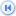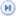Spike's Calculators

# Time

Time units table. Conversion factors for standard units of time, including hours, minutes, seconds, weeks, days, years and more!

one year (yr)0.01centuries (c.)one century (c.) =100years (yr)
one year (yr)1years (yr)one year (yr) = 1years (yr)
one year (yr)12months (mo)one month (mo) =0.0833333333333333years (yr)
one year (yr)52.1428571428571weeks (wk)one week (wk) =0.0191780821917808years (yr)
one year (yr)365days (d)one day (d)=0.00273972602739726years (yr)
one year (yr)8760hours (hr)one hour (hr) =0.000114155251141553years (yr)
one year (yr)525600minutes (min)one minute (min) = 0.00000190258751902588years (yr)
one year (yr)31536000seconds (sec)one second (sec) = 0.0000000317097919837646years (yr)
one year (yr)31536000000milliseconds (ms)one millisecond (ms) =0.0000000000317097919837646years (yr)
one year (yr)31536000000000microseconds (µs)one microsecond (µs) =0.0000000000000317097919837646years (yr)
one year (yr)31536000000000000nanoseconds (ns)one nanosecond (ns) =0.000000000000000031709791983765years (yr)
one century (c.) =1centuries (c.)one century (c.) =1centuries (c.)
one century (c.) =100years (yr)one year (yr) = 0.01centuries (c.)
one century (c.) =1200months (mo)one month (mo) =0.000833333333333333centuries (c.)
one century (c.) =5214.28571428571weeks (wk)one week (wk) =0.000191780821917808centuries (c.)
one century (c.) =36500days (d)one day (d)=0.0000273972602739726centuries (c.)
one century (c.) =876000hours (hr)one hour (hr) =0.00000114155251141553centuries (c.)
one century (c.) =52560000minutes (min)one minute (min) = 0.0000000190258751902588centuries (c.)
one century (c.) =3153600000seconds (sec)one second (sec) = 0.000000000317097919837646centuries (c.)
one century (c.) =3153600000000milliseconds (ms)one millisecond (ms) =0.000000000000317097919837646centuries (c.)
one century (c.) =3153600000000000microseconds (µs)one microsecond (µs) =0.000000000000000317097919837646centuries (c.)
one century (c.) =3153600000000000000nanoseconds (ns)one nanosecond (ns) =0.000000000000000000317097919838centuries (c.)
one month (mo) = 0.000833333333333333centuries (c.)one century (c.) =1200months (mo)
one month (mo) = 0.0833333333333333years (yr)one year (yr) = 12months (mo)
one month (mo) = 1months (mo)one month (mo) =1months (mo)
one month (mo) = 4.34523809523809weeks (wk)one week (wk) =0.23013698630137months (mo)
one month (mo) = 30.4166666666667days (d)one day (d)=0.0328767123287671months (mo)
one month (mo) = 730hours (hr)one hour (hr) =0.00136986301369863months (mo)
one month (mo) = 43800minutes (min)one minute (min) = 0.0000228310502283105months (mo)
one month (mo) = 2628000seconds (sec)one second (sec) = 0.000000380517503805175months (mo)
one month (mo) = 2628000000milliseconds (ms)one millisecond (ms) =0.000000000380517503805175months (mo)
one month (mo) = 2628000000000microseconds (µs)one microsecond (µs) =0.000000000000380517503805175months (mo)
one month (mo) = 2628000000000000nanoseconds (ns)one nanosecond (ns) =0.000000000000000380517503805175months (mo)
one week (wk) =0.000191780821917808centuries (c.)one century (c.) =5214.28571428571weeks (wk)
one week (wk) =0.0191780821917808years (yr)one year (yr) = 52.1428571428571weeks (wk)
one week (wk) =0.23013698630137months (mo)one month (mo) =4.34523809523809weeks (wk)
one week (wk) =1weeks (wk)one week (wk) =1weeks (wk)
one week (wk) =7days (d)one day (d)=0.142857142857143weeks (wk)
one week (wk) =168hours (hr)one hour (hr) =0.00595238095238095weeks (wk)
one week (wk) =10080minutes (min)one minute (min) = 0.0000992063492063491weeks (wk)
one week (wk) =604800seconds (sec)one second (sec) = 0.00000165343915343915weeks (wk)
one week (wk) =604800000milliseconds (ms)one millisecond (ms) =0.00000000165343915343915weeks (wk)
one week (wk) =604800000000microseconds (µs)one microsecond (µs) =0.00000000000165343915343915weeks (wk)
one week (wk) =604800000000000nanoseconds (ns)one nanosecond (ns) =0.00000000000000165343915343915weeks (wk)
one day (d) =0.0000273972602739726centuries (c.)one century (c.) =36500days (d)
one day (d) =0.00273972602739726years (yr)one year (yr) = 365days (d)
one day (d) =0.0328767123287671months (mo)one month (mo) =30.4166666666667days (d)
one day (d) =0.142857142857143weeks (wk)one week (wk) =7days (d)
one day (d) =1days (d)one day (d)=1days (d)
one day (d) =24hours (hr)one hour (hr) =0.0416666666666667days (d)
one day (d) =1440minutes (min)one minute (min) = 0.000694444444444444days (d)
one day (d) =86400seconds (sec)one second (sec) = 0.0000115740740740741days (d)
one day (d) =86400000milliseconds (ms)one millisecond (ms) =0.0000000115740740740741days (d)
one day (d) =86400000000microseconds (µs)one microsecond (µs) =0.0000000000115740740740741days (d)
one day (d) =86400000000000nanoseconds (ns)one nanosecond (ns) =0.0000000000000115740740740741days (d)
one hour (hr) =0.00000114155251141553centuries (c.)one century (c.) =876000hours (hr)
one hour (hr) =0.000114155251141553years (yr)one year (yr) = 8760hours (hr)
one hour (hr) =0.00136986301369863months (mo)one month (mo) =730hours (hr)
one hour (hr) =0.00595238095238095weeks (wk)one week (wk) =168hours (hr)
one hour (hr) =0.0416666666666667days (d)one day (d)=24hours (hr)
one hour (hr) =1hours (hr)one hour (hr) =1hours (hr)
one hour (hr) =60minutes (min)one minute (min) = 0.0166666666666667hours (hr)
one hour (hr) =3600seconds (sec)one second (sec) = 0.000277777777777778hours (hr)
one hour (hr) =3600000milliseconds (ms)one millisecond (ms) =0.000000277777777777778hours (hr)
one hour (hr) =3600000000microseconds (µs)one microsecond (µs) =0.000000000277777777777778hours (hr)
one hour (hr) =3600000000000nanoseconds (ns)one nanosecond (ns) =0.000000000000277777777777778hours (hr)
one minute (min) =0.0000000190258751902588centuries (c.)one century (c.) =52560000minutes (min)
one minute (min) =0.00000190258751902588years (yr)one year (yr) = 525600minutes (min)
one minute (min) =0.0000228310502283105months (mo)one month (mo) =43800minutes (min)
one minute (min) =0.0000992063492063491weeks (wk)one week (wk) =10080minutes (min)
one minute (min) =0.000694444days (d)one day (d)=1440minutes (min)
one minute (min) =0.016666667hours (hr)one hour (hr) =60minutes (min)
one minute (min) =1minutes (min)one minute (min) = 1minutes (min)
one minute (min) =60seconds (sec)one second (sec) = 0.0166666666666667minutes (min)
one minute (min) =60000milliseconds (ms)one millisecond (ms) =0.0000166666666666667minutes (min)
one minute (min) =60000000microseconds (µs)one microsecond (µs) =0.0000000166666666666667minutes (min)
one minute (min) =60000000000nanoseconds (ns)one nanosecond (ns) =0.0000000000166666666666667minutes (min)
one second (sec) =0.000000000317097919837646centuries (c.)one century (c.) =3153600000seconds (sec)
one second (sec) =0.0000000317097919837646years (yr)one year (yr) = 31536000seconds (sec)
one second (sec) =0.000000380517503805175months (mo)one month (mo) =2628000seconds (sec)
one second (sec) =0.00000165343915343915weeks (wk)one week (wk) =604800seconds (sec)
one second (sec) =0.0000115740740740741days (d)one day (d)=86400seconds (sec)
one second (sec) =0.000277778hours (hr)one hour (hr) =3600seconds (sec)
one second (sec) =0.016666667minutes (min)one minute (min) = 60seconds (sec)
one second (sec) =1seconds (sec)one second (sec) = 1seconds (sec)
one second (sec) =1000milliseconds (ms)one millisecond (ms) =0.001seconds (sec)
one second (sec) =1000000microseconds (µs)one microsecond (µs) =0.000001seconds (sec)
one second (sec) =1000000000nanoseconds (ns)one nanosecond (ns) =0.000000001seconds (sec)
one millisecond (ms)0.000000000000317097919837646centuries (c.)one century (c.) =3153600000000milliseconds (ms)
one millisecond (ms)0.0000000000317097919837646years (yr)one year (yr) = 31536000000milliseconds (ms)
one millisecond (ms)0.000000000380517503805175months (mo)one month (mo) =2628000000milliseconds (ms)
one millisecond (ms)0.00000000165343915343915weeks (wk)one week (wk) =604800000milliseconds (ms)
one millisecond (ms)0.0000000115740740740741days (d)one day (d)=86400000milliseconds (ms)
one millisecond (ms)0.000000277777777777778hours (hr)one hour (hr) =3600000milliseconds (ms)
one millisecond (ms)0.0000166666666666667minutes (min)one minute (min) = 60000milliseconds (ms)
one millisecond (ms)0.001seconds (sec)one second (sec) = 1000milliseconds (ms)
one millisecond (ms)1milliseconds (ms)one millisecond (ms) =1milliseconds (ms)
one millisecond (ms)1000microseconds (µs)one microsecond (µs) =0.001milliseconds (ms)
one millisecond (ms)1000000nanoseconds (ns)one nanosecond (ns) =0.000001milliseconds (ms)
one microsecond (µs) =0.00000000000000317097919837646years (yr)one year (yr) = 315360000000000microseconds (µs)
one microsecond (µs) =0.0000000000000317097919837646months (mo)one month (mo) =31536000000000microseconds (µs)
one microsecond (µs) =0.000000000000380517503805175weeks (wk)one week (wk) =2628000000000microseconds (µs)
one microsecond (µs) =0.00000000000165343915343915days (d)one day (d)=604800000000microseconds (µs)
one microsecond (µs) =0.0000000000115740740740741hours (hr)one hour (hr) =86400000000microseconds (µs)
one microsecond (µs) =0.000000000277777777777778minutes (min)one minute (min) = 3600000000microseconds (µs)
one microsecond (µs) =0.0000000166666666666667seconds (sec)one second (sec) = 60000000microseconds (µs)
one microsecond (µs) =0.000001milliseconds (ms)one millisecond (ms) =1000000microseconds (µs)
one microsecond (µs) =0.001microseconds (µs)one microsecond (µs) =1000microseconds (µs)
one microsecond (µs) =1nanoseconds (ns)one nanosecond (ns) =1microseconds (µs)
one nanosecond (ns) =0.000000000000000000317097919838centuries (c.)one century (c.) =3153600000000000000nanoseconds (ns)
one nanosecond (ns) =0.000000000000000031709791983765years (yr)one year (yr) = 31536000000000000nanoseconds (ns)
one nanosecond (ns) =0.000000000000000380517503805175months (mo)one month (mo) =2628000000000000nanoseconds (ns)
one nanosecond (ns) =0.00000000000000165343915343915weeks (wk)one week (wk) =604800000000000nanoseconds (ns)
one nanosecond (ns) =0.0000000000000115740740740741days (d)one day (d)=86400000000000nanoseconds (ns)
one nanosecond (ns) =0.000000000000277777777777778hours (hr)one hour (hr) =3600000000000nanoseconds (ns)
one nanosecond (ns) =0.00000000001666666666666670minutes (min)one minute (min) = 60000000000nanoseconds (ns)
one nanosecond (ns) =0.000000001seconds (sec)one second (sec) = 1000000000nanoseconds (ns)
one nanosecond (ns) =0.000001milliseconds (ms)one millisecond (ms) =1000000nanoseconds (ns)
one nanosecond (ns) =0.001microseconds (µs)one microsecond (µs) =1000nanoseconds (ns)
one nanosecond (ns) =1nanoseconds (ns)one nanosecond (ns) =1nanoseconds (ns)